International Journal of Energy and Power Engineering
Volume 5, Issue 1-1, February 2016, Pages: 65-74

Research on Magnetostriction Property of Silicon Steel Sheets

Yanli Zhang, Yuandi Wang, Dianhai Zhang, Ziyan Ren, Dexin Xie

School of Electrical Engineering, Shenyang University of Technology, Shenyang, China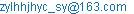(Yanli Zhang)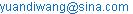(Yuandi Wang)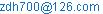(Dianhai Zhang)(Ziyan Ren)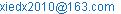(Dexin Xie)

Yanli Zhang, Yuandi Wang, Dianhai Zhang, Ziyan Ren, Dexin Xie. Research on Magnetostriction Property of Silicon Steel Sheets. International Journal of Energy and Power Engineering. Special Issue: Numerical Analysis, Material Modeling and Validation for Magnetic Losses in Electromagnetic Devices. Vol. 5, No. 1-1, 2015, pp. 65-74. doi: 10.11648/j.ijepe.s.2016050101.20

Abstract: When electrical devices such as electrical machines and transformers are in operation, the magnetostriction of the silicon steel sheets results in the deformation of the laminated iron core, which aggravates the vibration and noise of the iron core. Therefore, it is of significance to study the relationship between the magnetostrictive effect and external magnetic field. The aim of this paper is to report the researches finished, in progress, and to be done by the authors’ group, so as to discuss the idea how to investigate the magnetostriction property of the silicon steel sheets, whether non-oriented or grain-oriented. Measurement method, property model formulation, and the application of the model to finite element analysis are described. The relative numerical results are given.

Keywords: Magnetostriction, Principal Strain, Anisotropic Property, Silicon Steel Sheet, Higher Harmonic Magnetic Field, DC Magnetic Bias, Finite Element Analysis

1. Introduction

Magnetostriction effect refers to the phenomenon that the size of ferromagnetic material at different directions elongates or contracts in supplied magnetic field, following the variation of the field. Silicon steel is a kind of typical ferromagnetic material. As a magnetic path silicon steel laminated iron core is used in electrical devices such as electrical machines, transformers, shunt reactors, etc. When these devices are in operation, the magnetostriction of the silicon steel sheets results in the deformation of the iron core, which aggravates its vibration and noise. The larger the product rating capacity is, the more obvious the effect is. In recent years, the capacity of power transformers and electric machines increases rapidly. As an example, the power transformers with their rating capacity and voltage up to 1000MVA and 1000kV have been put into operation. Although electrical devices have passed the delivery test standard in factory, the vibration and noise of some of the devices may excess the standard often in site. The reason is that the delivery test is carried out in standard test conditions, while certain complicated factors, involving DC magnetic bias, higher harmonics or overload do not be included in the test standard. Otherwise, these abnormal factors are difficult to be included in test conditions. Therefore, it is necessary to consider and analyze carefully the vibration and noise caused by magnetostriction of the silicon steel laminated core in the design process of the electrical devices with large scale, and to seize the mechanism and regularity of the magnetostriction property of the silicon steel sheet, thus to find the way of reducing the vibration and noise.

In fact, many papers have been published on decreasing the vibration and noise. However, it still needs to go deep into the research in this respect due to the complexity of the problem. The authors of this paper intend to report the researches finished, in progress, and will be done by the authors’ group, to discuss the idea how to investigate the magnetostriction property of the silicon steel sheets, whether non-oriented or grain-oriented.

2. Measurement and Simulation of Magnetostriction Property of Silicon Steel Sheets Under Standard Magnetizing Forms

In general, research for magnetostriction property of the silicon steel sheets includes property measurement, property model formulation, and coupling the property model with Finite Element (FE) analysis.

Tracing the researches of recent years, it is known that the magnetostriction property measurement of silicon steel sheets is developing from one dimensional (1D) to two dimensional (2D) measuring. The 1D measurement refers to the strain testing under alternating magnetization, i.e., the direction of magnetic field in one time period varies along a straight line, and only the strains at the magnetizing direction are measured, without regard to the intersection angle between the strain and magnetic flux density. For the measuring technique of anisotropic magnetostriction property, the research is just in initial stage. The 2D measurement means that the measuring is carried out under rotating magnetic field, which is supplied along the two directions perpendicular to each other, and the directions of the flux density can be controlled to be changed periodically. The 1D single sheet magnetostriction test system has been used extensively, and an international standard technical report, IEC/TR 62581, was issued at 2010 . About the 2D measurement, reference  and  (Somkun SAnthony J. Moses et al., 2010 and 2012) report the test technique and show related measuring results, while references [4-6] (M. Enokizono et al., 2011-1012) apply similar test method to measure the 2D magnetostriction property and research further the property under external stress. Constructing the 2D test system is a complicated task, so that the measurement and research are still in the stage of laboratory.

This section describes the authors’ study on anisotropic magnetostriction property of silicon steel sheets with 1D magnetostriction test system firstly, then the numerical formulation of the model for the magnetostriction property and the relationship between the principal strain and the direction of magnetization using the triaxia1 measuring method[7-10], finally the primary 2D magnetostriction property measurement.

2.1. Study on Anisotropic Magnetostriction Property of Silicon Steel Sheets with 1D Measuring EquipmentFigure 1. Magnetostrictive measurement device.

Figure 1 is a photo of traditional magnetostrictive measurement device MST500 for single silicon steel sheet specimen. The magnetostriction test unit consists of laser sender, receiver, and reflector. The laser sender emits light beam onto the reflector, and the reflector sends the beam back to the laser to test the infinitesimal displacement of the specimen. An external magnetic field is supplied along the longitudinal direction of the specimen. The advantages of the traditional device are as follows:

1)  The laser sensor is with high resolution up to 10 nm/m.

2)  It can provide the global magnetostrictive effect, while the traditional test by strain gauge only provides local deformation of the specimen.

3)  The size of the specimen is a long strip of 500 mm´100 mm. Compared with that of 60 mm´60 mm in 2D magnetostriction test system, the influence of shear force in processing the specimen on the magnetostriction property can be reduced.

Considering that the test system can provide the magnetic field and test the strain only in the longitudinal direction, the specimens are cut in different angle, making their longitudinal direction at an angle with the rolling direction (RD) range from 0o to 90o with the interval of 15o to investigate the magnetostrictive property at different directions.

The alternating magnetic field of 50 Hz is created by the exciting winding, and the non-oriented silicon steel specimens are tested. The time-varying strain of the specimen is obtained with the laser sender and receiver device. Fig.2 (a) and (b) show the magnetostrictive strain curves along different magnetized directions in polar coordinate for the magnetic flux density of 0.6T and 1.4T respectively. It can be seen that the magnetostriction property of non-oriented silicon steel is also anisotropic, and the strain is related with not only the magnitude of magnetic flux density, but also its angle with RD.

To formulate the anisotropic magnetostrictive property, the conventional method uses the characteristic curves in RD and the transverse direction (TD), that is, expresses the linear magnetic pressure equation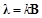(1)

As,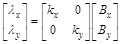(2)

further. This formulation could be named orthogonal magnetostriction model. That means the magnetostriction property at arbitrary direction can be obtained by simplified computation using the property at RD and TD. At present, some commercial software is just based on this model to describe magnetostriction property of material.

However, the testing results shown in Fig.2 indicate that the orthogonal model is too simple to express the magnetostriction property at arbitrary direction. To improve the model, corresponding to the test method described in this section, the formulation of magnetostriction property of silicon steel sheet at arbitrary direction can be given as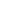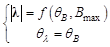(3)

where the magnitude of the strain vector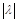is the peak-to-peak value of the magnetostriction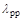, and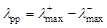(4)

in which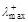and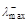are the maximal elongation and contraction strain respectively;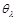and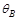are the angles of the strain vector and magnetic flux density vector with the RD respectively.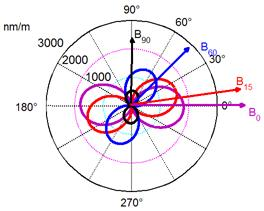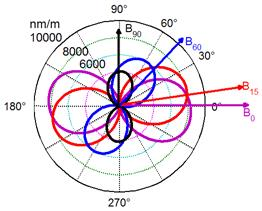(a)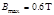(b)Figure 2. Magnetostrictive strain curves along different magnetized directions in polar coordinate.

2.2. Measurement of Principal Strain and Improvement of Magnetostriction ModelFigure 3. Illustration of measurement device for triaxial laser method(Top view).

It is known that from equation (3) the angles of strain and magnetic flux density,and, are equal to each other. This is restricted by the test method described in Section 2.1, because the obtained magnetostriction is just the strain at the direction of magnetization, and not the principal strain. To acquire the magnetostriction principal strain of silicon steel sheet the "triaxial laser method" is used here, referring the triaxial strain gauge test technique in 2D magnetostriction measuring system. The method can be described as follows. At first, set the three test directions, making the angle between the directions and the longitudinal direction of the specimen as 0o, 45o, and 90o, as shown with the location a, location b and location c in Fig. 3. Then put the laser and reflector on the three directions in turn, and measure the strains at each direction in one magnetizing period. After that, based on the knowledge of plane strain in material mechanics, substitute the measured strains la, lb, and lc into (5) given as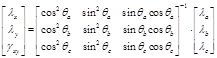(5)

to calculate the orthogonal line strains lx, ly and a shear strain gxy. Finally, calculate the maximal principal strain l and the direction ql at which the l occurs according to (6) and (7) given as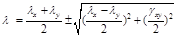(6)(7)

The specimens are still cut at different angles making the longitudinal directions with the RD range from 0o to 90 o with the interval of 15o. Fig. 4 illustrates the 3 magnetostrictive loops of a specimen of 45o at the 3 test directions when the alternating magnetic field varying from 0.6T to 1.6T. The loops also called butterfly diagram. Contrasting the Fig. 4 (a), (b) and (c), it can be seen that the magnetostrictive pattern of the specimens at different directions under alternating magnetization is quite different.

The variation of the principal strain magnitude and direction along with the varying of the supplied magnetic field can be calculated using the test data of Fig. 4 combined with (6) and (7). Fig. 5 describes the magnetostriction principal strain magnitude and direction versus the magnetic flux density. Compared with Fig. 4, it can be seen that the magnetostriction is the largest one at the direction of principal strain, and the direction will change along with the variation of the magnetization. These results are consistent with theoretical analysis.

The magnitude and direction of the principal strain versus that of magnetic flux density are given in Fig. 6, from which we can see that the principal strain increases gradually along with the magnetic flux density going up, and when the flux density reaches to 1.6T the magnetostriction tends to saturation.  It is also seen that from Fig. 6(b) for a certain direction of magnetization, the direction of principal strainchanges with the increase of magnetic flux density. The curve ofis relative gentle nearof 0°and 90°, where principal strain direction is near the magnetostriction direction. That means when the silicon steel sheet is magnetized at the RD or TD, the maximal magnetostriction deformation occurs just at the magnetized direction, while the deformation deviates the magnetization direction whenequals other angles. Therefore, the magnetostriction deformation at the magnetization direction given by using the conventional method is not the maximal deformation, which cannot truly reflect the magnetostriction effect of silicon steel sheets.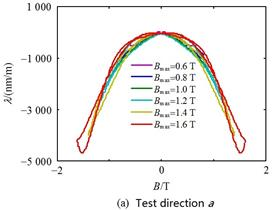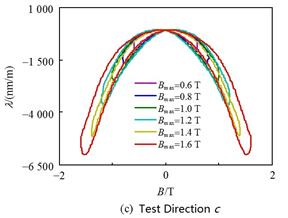Figure 4. Magnetostrictive loops along three detections.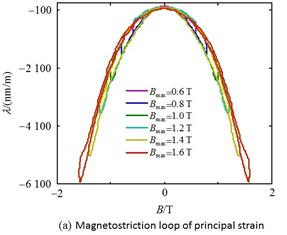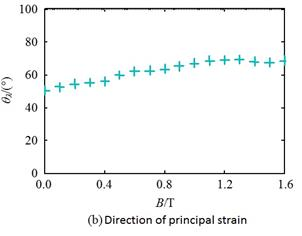Figure 5. Principal strain of magnetostriction.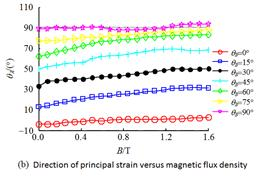Figure 6. Relationship curve between principal strain and magnetic flux density.

To sum up, the magnetostriction property of silicon steel sheets is vector-anisotropic, and the principal strain vector relates to not only the magnitude, but also the direction of magnetic field where the sheet locates in. Therefore, the vectorial magnetostriction property of silicon steel sheets expressed by principal strain has to be given as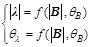(8)

Equation (8) is an improved formulation to (3), where |l| is the magnitude of principal strain, which is the function of magnetic flux density and its direction angle qB; same as its magnitude, the direction angle of the principal strain ql is also the function of magnetic flux density vector.

To incorporate the magnetostriction property model into FE analysis, the concrete computation steps are sketched now as follows:

Ÿ According to the relationship of principal strain vector and magnetic flux density vector described in (8), a three dimensional curved surface to depict the relation of the two vectors is obtained by using the B-spline function interpolation of measured data of Fig. 6, as illustrated in Fig. 7.

Ÿ Calculate the magnetic flux density,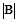andof each element by electromagnetic field FE analysis software, then find the magnetostriction principal strain of each element,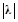andbased on the curve surface of Fig. 7 with interpolation. Finally, the distribution of the principal strain in whole computing region is obtained.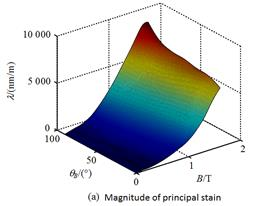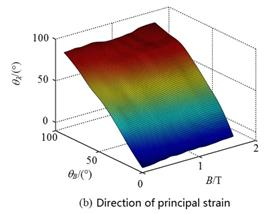Figure 7. Curve surface of vector relationship between principal strain and magnetic flux density.

It is noted that the computational method above described is an indirect coupling one, and because that the deformation caused by the magnetostriction is too small to effect the whole structure of the analysed device, iterative calculation does not be needed.

2.3. Measurement of 2D Magnetostriction Property of Silicon Steel Sheets in the Condition of Rotational Magnetization

The measurement of magnetostriction described in Section 2.1 and 2.2 is carried out under alternating magnetization. However, in practical operation of electrical machines and transformers there exist local rotating magnetic fields, i.e., the situation that the local magnetic flux density vector changes its direction continually in a period. Therefore, the 2D magnetostriction property of silicon steel sheets has to be studied. This is the research we are doing now, but from the initial test results some enlightenment could be also gained.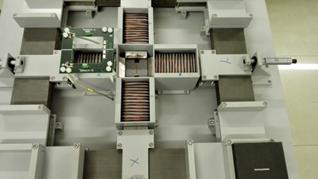Figure 8. 2D Magnetic field generator.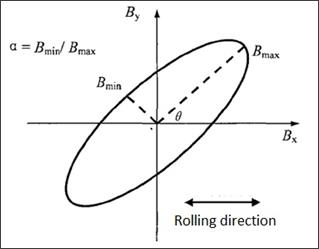Figure 9. The ellipse created by the rotation of B vector in a time period.

Fig. 8 is the magnetic field generator for 2D magnetostriction property measurement. Its main magnetic circuit is the same as that of 2D magnetic property (B versus H) tester, which is used by some researchers in recent years. In the two exciting windings which are perpendicular to each other, the sinusoidal time-varying electric currents are controlled to produce a rotating magnetic field. The vertex locus of magnetic flux density vector in a period is an ellipse, see Fig. 9. Now express the components of the magnetic flux density, Bx and By as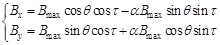(9)

where Bmax is the length of the ellipse long axis,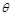is the angle between the long axis and RD of the specimen,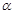is the ratio of the long axis to the minor axis,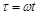. The ellipse locus is controlled by changing the three parameters Bmax,, and. The silicon steel sheet of 60mm×60mm locates in the center of the main magnetic path. The triaxial strain gauge test method described in Section 2.2 is still used for measuring the strain in the directions of 0o45o and 90o (see Fig. 3) . The principal strain is calculated according to (6) and (7). Fig. 10 illustrates the sketch map of the strain gauges.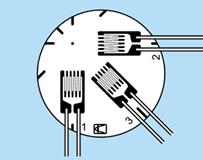Figure 10. Structure sketch map of strain gauge.

The magnetostriction property of non-oriented silicon steel sheets with different Bmax,, andis measured by the author’s group. Fig. 11 shows the loci of principal strain and magnetic flux density B in a time period for Bmax=1.0 T,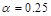andequals 15°, 45° and 75° respectively, where the black dotted line stands for the ellipse locus of B, red solid line for the locus of principal strain, blue arrow is for the long axis of the ellipse, and black arrow for the direction of the maximum principal strain. From the figure it can be seen that there are an intersection between the two arrows. A lot of tests indicate that the maximal principal strain vector is the function of the magnetic flux density magnitude, angle, and the ratio of ellipse long axis to minor axis. Therefore, more variables are introduced for the 2D magnetostrictive property in the condition of rotating magnetization. The measuring technique and model formulation are more complicated compared with the case of 1D magnetostrictive property. The further research is now under way.

3. Measurement and Simulation of Magnetostriction Property of Silicon Steel Sheets Under Abnormal Magnetizing Forms

3.1. Influence of Magnetic Field Harmonic Components on Magnetostriction and Noise 

There exist higher harmonic components in exciting voltage of electrical machines and transformers in practical operation due to the nonlinear loads in power system. The existence of the harmonics not only increase the additional losses, but also change the magnetostriction property of silicon steel sheets and intensify the vibration and noise of iron core. Therefore, it is significant to investigate the magnetostriction property of silicon steel sheets under the magnetic field with higher harmonics.

The authors’ group measured the magnetostrictive waveforms of non-oriented grain electrical steel sheet by means of controlling the magnitude and phase angle of the third harmonic of magnetic field. Considering the third harmonic is the major higher harmonic in the resultant magnetic field, the magnetic flux density is expressed as,(10)

where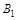and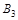are the amplitude of fundamental and the third harmonic magnetic flux density, θ3 is the phase angle of the third harmonic, ω = 2πff = 50Hz. Different supplied magnetic field can be obtained by changing,and θ3. The measuring results indicate that in addition to the second harmonic (f = 100Hz), which account for the major component, the fourth (f = 200Hz) and the sixth (f = 300Hz) harmonic also occupy a certain proportion of the magnetostriction, so that the influence of the harmonic magnetic field on the fourth and the sixth harmonic of magnetostriction is discussed.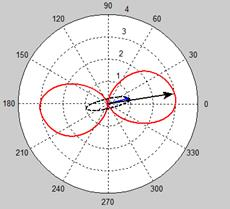(a)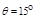.                                    (b)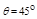.(c)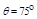.

Figure 11. Loci of magnetostriction principal strainand magnetic flux density.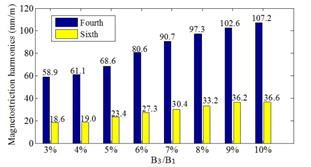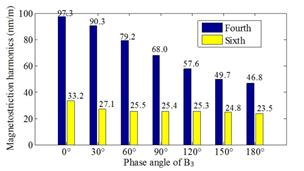(a) Effect of the third harmonic magnetic field proportion.         (b) Effect of the third harmonic magnetic field phase angle.

Figure 12. Variation of magnetostrictive harmonic components with the increase of resultant magnetic flux when B = 1.0T.

The variation of magnetostrictive harmonic components with the increase of the resultant magnetic flux when B = 1.0T is investigated. The results are illustrated in Fig. 12. It is seen that along with the increase of the third harmonic component of magnetic field the magnetostrictive harmonic components also increase obviously, while the increase of the third harmonic phase angle of the magnetic field makes the magnetostrictive harmonic components reduced, especially for the fourth harmonic of magnetostriction. The measured relation curve surface is shown in Fig. 13.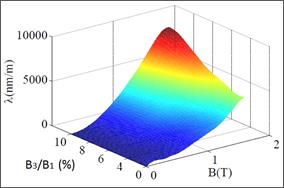Figure 13. Relationship between magnetostriction and magnetic flux density with the 3rd harmonic.

To find the noise due to the magnetostraction in the magnetic field with the third harmonic included, firstly solve the electromagnetic field in electrical device with a FE analysis software and find the waveform of element magnetic flux density, then carry out the Fourier analysis and reserve the amplitude of the fundamental and the third harmonic of element magnetic flux densities. After that, get the magnetostriction principal strain distribution under the resultant magnetic field by interpolating the relation curve surface of Fig. 13. Finally the expression of the so-called A-weighted velocity level (AWV)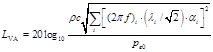(10)

is used to calculate the decibel (DB) value LVA of the noise, where ρ is the air density at room temperature, f is the fundamental frequency,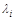is the i-th component of the principal strain,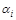is the corresponding AWV coefficient,is the minimal audible pressure. Figure 14 shows the noise caused by magnetostriction under the magnetic field with higher harmonic, it can be applied to get the distribution of noise in the electrical device by interpolation.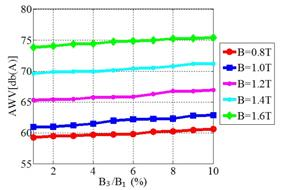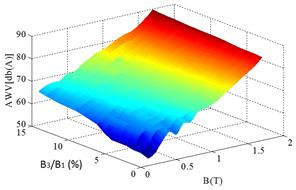(a) Effectof the third harmonic magnetic field proportion.         (b) Curve surface of noise versus harmonic magnetic field proportion.

Figure 14. Noise caused by magnetostriction under higher harmonics of magnetic field.

3.2. Influence of DC Bias Magnetic Field on Magnetostriction Property 

The external magnetic field may cover DC component when power transformers are in operation. It is necessary to identify how different magnetization patterns contribute to the magnetostriction noise of the iron cores. To measure the effect of the DC bias magnetic field on magnetostriction and noise, a DC component is superposed in the electric current of the exciting winding to create a DC bias magnetic field along the rolling or transverse direction of a grain-oriented silicon steel sheet. Using the method described in Section 2, the relationship of magnetostriction and magnetic field can be measured. Fig. 15 shows the waveforms of magnetic flux density and principal strain in a magnetization period along RD and TD when DC biased magnetic field intensity H = 30 A/m for B = 0.7, 0.9, 1.2 and 1.4T. It is worth noting that the waveform of magnetic flux density B is still sinusoidal time-varying without the DC component, shown as in Fig. 15(a) although the DC magnetic component exists, because that the B-testing coil is winded in the test specimen and the DC magnetic field component cannot induce voltage in the secondary coil. From Fig. 15(b) it can be seen that the principal strain of RD and TD increases obviously along with the increase of the magnetization. To illustrate the effect of DC bias on principal strain further, the comparison of principal strain of a GO silicon steel sheet with alternating B of 1.4T under different DC biased magnetic field H = 0, 10, 20, and 30 A/m along RD. It is seen that compared the situation without the DC component, the waveform of principal strain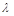with the DC bias is distorted and its waveform in the first half of period is different from that in the second half of period any more. That means the waveform of theincludes higher harmonic components in addition to the basic component of f = 100 Hz. Furthermore, with the increase of biased field supplied, the contractive principal strain along the RD in Fig. 16 (a) increases slightly, while the elongation strain along the TD in Fig. 16 (b) is obviously bigger than that without DC biased field. More studies to the measured data shown in Fig. 16 indicate that the presence of a DC biased magnetic field not only increases the amplitude of the magnetostrictive strain, but also changes the property of the principal strain from contractive characteristic to elongate one.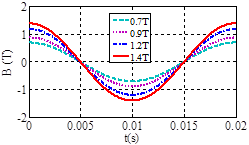(a) B versus t.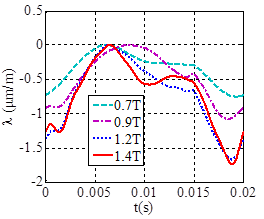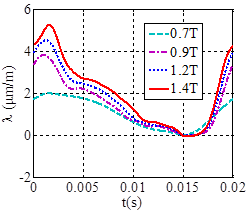(b) Principal strain along the RD.                     (c) Principal strain along the TD.

Figure 15. Measured magnetic flux density and principal strain waveform in one magnetization period under 30A/m biased field applied along the RD in a GO silicon steel sheet.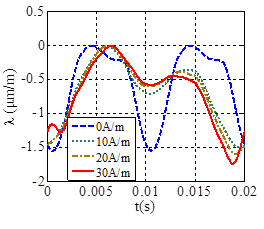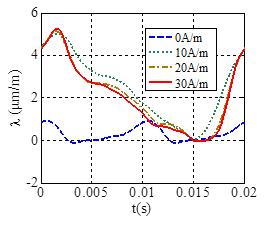(a) Principal strain along the RD.                                (b) Principal strain along the TD.

Figure 16. Principal strain under different DC biased field in a GO silicon steel sheet with alternating B of 1.4 T.

4. Conclusions

This paper introduces the research situation on magnetostriction property of silicon steel sheets in recent years initially, then mainly focuses the studies on this area by the author’s group.

The research on anisotropic magnetostrictive property of silicon steel sheets with 1D measuring equipment under alternating supplied magnetic field is described firstly, and the triaxial laser test method is used for measuring and calculating the principal strain. It can be concluded that the principal strain of silicon steel sheet, whether non-oriented or grain-oriented, have to be expressed by a vector with anisotropic characteristic, of which both the magnitude and direction angle are related with that of magnetic flux density vector. An improved magnetostrictive property model is proposed.

For the 2D magnetostrictive property of silicon steel sheets in the condition of rotational magnetization, some primary measured results are given to indicate the complexity of the property.

Otherwise, the simulation method combined the magnetostrictive property model and FE analysis is presented with its application to the research of the effect of the higher harmonics or DC bias magnetic field on magnetostriction and relative noise.

In fact, more researches need to be done on the magnetostrictive property of silicon steel sheets, such as better formulation of the property model, direct couple method of the model with FE analysis, and further research of the 2D magnetostrictive property under rotating external magnetic field.

References

1. InternationalElectrotechnical Commission.IEC/TR62581 Electrical Steel–Methods of measurement of the magnetostriction characteristics by means of single sheet and Epstein test specimens[S].Switzerland:IEC Central Office,2010.
2. SomkunS,Moses A J,Anderson P I,et al.Magnetostriction anisotropy and rotational magnetostriction of a nonoriented electrical steel.IEEE Transactions on Magnetics,2010,46(2):302-305.
3. Somkun S,Moses A J,Anderson P I.Measurement and modeling of 2-D magnetostriction of nonoriented electrical steel [J].IEEE Transactions on Magnetics,2012,48(2):711-714.
4. Kai Y,Todaka T,Enokizono M,et al.Measurement of the two-dimensional magnetostriction and the vector magnetic property for a non-oriented electrical steel Sheet under stress.Journal of Applied Physics,2012,111(7):07E320-1-07E320-3.
5. Daisuke W, Takashi T,Masato E.Three-dimensional magnetostriction and vector magnetic properties under alternating magnetic flux conditions in arbitrary direction.Electrical engineering in Japan,2012,179(4):1-9.
6. Daisuke W,Takashi T,Masato E.Measurement of three-dimensional magnetostriction on grain oriented electrical steel sheet [J].Journal of Electrical Engineering,2011,62(3):153-157.
7. Yanli Zhang, Xiaoguang Sun, Dexin Xie, et al. Measurement and simulation of magnetostrictive properties for non-grain oriented electrical steel sheet. Transactions of China Electrotechnical Society, 2013, 28(11): 176-181. (in Chinese).
8. Yanli Zhang, Xiaoguang Sun, Dexin Xie, et al. Modeling of anisotropic magnetostriction property of non-oriented silicon steel sheet. Proceedings of the CSEE, 2014,34(27):4731-4736. (in Chinese).
9. Yanli Zhang, Jiayin Wang, Xiaoguang Sun, et al. Measurement and modeling of anisotropic magnetostriction characteristic of grain-oriented silicon steel sheet under DC bias. IEEE Transactions on Magnetics, 2014,50(2):7008804.
10. YanliZhang, Yangyang Wang, Dianhai Zhang, Ziyan Ren, Dexin Xie, Baodong Bai, Jiakuan Xia. Vector MagnetostrictivePropertiesof Electrical Steel Sheet With Alternating Magnetization, to be published inProceedings of the CSEE, 2015. (in Chinese).
11. Yanli Zhang, Qiang Li, Yangyang Wang, Dianhai Zhang, Ziyan Ren, Baodong Bai, Dexin Xie.Analysis on Magnetostrictive Properties of Silicon Steel Sheet UnderHarmonic Magnetic Field.Transactions of China Electrotechnical Society, 2015, 30(14): 544-549. (in Chinese).
12. Yanli Zhang, Qiang Li, Dianhai Zhang, Baodong Bai, Dexin Xie, and Chang Seop Koh. Magnetostriction ofsiliconsteelsheetsunderdifferentmagnetizationconditions.IEEE Transactions on Magnetics, 2015,51(11):6101604.

 Contents 1. 2. 2.1. 2.2. 2.3. 3. 3.1. 3.2. 4.
Article ToolsAbstractPDF(1020K)